Theory of Columns

# Theory of Columns Notes | Study Mechanical Engineering SSC JE (Technical) - Mechanical Engineering

## Document Description: Theory of Columns for Mechanical Engineering 2022 is part of Strength of Materials (SOM) for Mechanical Engineering SSC JE (Technical) preparation. The notes and questions for Theory of Columns have been prepared according to the Mechanical Engineering exam syllabus. Information about Theory of Columns covers topics like and Theory of Columns Example, for Mechanical Engineering 2022 Exam. Find important definitions, questions, notes, meanings, examples, exercises and tests below for Theory of Columns.

Introduction of Theory of Columns in English is available as part of our Mechanical Engineering SSC JE (Technical) for Mechanical Engineering & Theory of Columns in Hindi for Mechanical Engineering SSC JE (Technical) course. Download more important topics related with Strength of Materials (SOM), notes, lectures and mock test series for Mechanical Engineering Exam by signing up for free. Mechanical Engineering: Theory of Columns Notes | Study Mechanical Engineering SSC JE (Technical) - Mechanical Engineering
 1 Crore+ students have signed up on EduRev. Have you?

THEORY OF COLUMNS

Compression Member : A compression member is a structural member which is straight and subjected to two equal and opposite compressive forces applied at its ends.
Column: It is defined as a vertical member which is fixed at both the ends and subjected to an axial compressive load.Column, is a compression member that is so slender compared to its length that under gradually increasing loads, it fails by buckling at loads
considerably less that those required to cause failure by crushing.
• A compression member is generally considered to be a long column when its unsupported length is more than 10 times its least lateral dimension.
• Column, Stanchion or post is a vertical compression member supporting floors or girders in a building.
• Principal rafter is a top chord member in a roof truss.
• Boom is the principal compression member in a crane.
• Strut is commonly used for compression member in a roof truss. It may either be in vertical position or in inclined position.
MODES OF FAILURE OF A COLUMN
Elastic instability
A column subjected to axial load, may fail under the following modes:
(i) Crushing
(ii) Buckling
(iii) Mixed mode of Buckling and Crushing.
Euler's Theory (Buckling failure)

•  Assumption of Euler's theory:

(i) Axis of the column is perfectly straight when unloaded.
(ii) The line of thrust coincides exactly with the unstrained of the column.
(iii) Flexural rigidity EI is uniform
(iv) Material is isotropic and homogeneous.
(v) The buckling value of load is assumed to obtain for all degrees of flexure.
(vi) Length is assumed to be too large with respect to cross section.

•   Limitation of Euler's Formula:

(i) The critical load that causes buckling depends not on strength of the material, but only on its dimensions and modulus of elasticity. This converts the strut problem from the problem of instability to a problem of stress, of which Euler's theory takes no account.
(ii) In order for Euler's formula to be applicable, the critical stress p1 must not exceed the proportional limit.

IDEAL END CONSITION & EFFECTIVE LENGTH
Depending upon various combinations of restraints, there may be the following four cases of end condition:
Case 1 : Both ends hinged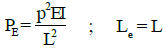Case 2 : One end fixed and other end free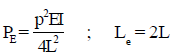Case 3 : Both ends fixed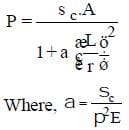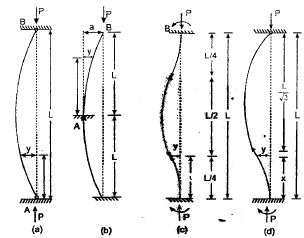PRACTICAL END CONSITION AND EFFECTIVE LENGTH FACTORS:
As per IS : 800 - 1984, following table can be used for finding effective length:
Effective length of compression members
Radius of Gyration and Slenderness Ratio:

•  The radius of gyration of a section is given by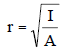where, I = Moment of Inertia
A = area of section

•  Rankine's formula

The Rankine's formula is applicable for all columns ranging form very short to very long struts.
Ranking proposed,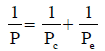Where, Pc = fc.A= ultimate load for a strut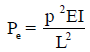= Euler crippling load for the standard case
After rearranging above relations,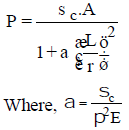= Rankine's constant for a particular material

The document Theory of Columns Notes | Study Mechanical Engineering SSC JE (Technical) - Mechanical Engineering is a part of the Mechanical Engineering Course Mechanical Engineering SSC JE (Technical).
All you need of Mechanical Engineering at this link: Mechanical Engineering

## Mechanical Engineering SSC JE (Technical)

6 videos|89 docs|55 tests
 Use Code STAYHOME200 and get INR 200 additional OFF

## Mechanical Engineering SSC JE (Technical)

6 videos|89 docs|55 tests

Track your progress, build streaks, highlight & save important lessons and more!

,

,

,

,

,

,

,

,

,

,

,

,

,

,

,

,

,

,

,

,

,

;